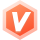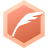# 5.8 汇编语言：汇编高效除法运算lyshark全栈领域优质创作者博客专家认证
2023-11-17 09:46:07

• 1.如果被除数是一个未知数，那么编译器无法确定数值，则编译器会使用原始的`div`指令计算，程序的执行效率会变低。
• 2.如果除数是2的次幂，那么可以将其转化为处理速度快的`shr`逻辑右移指令指令，该指令的执行只需要1个时钟周期，效率最高。
• 3.如果要进行2的次幂，并且该数是有符号数，则只需要使用`sar`算数右移指令，即可进行快速除法运算。

### 8.1 使用IDIV指令完成除法

• 计算除法时应遵循:
• 如果除数为`8位`被除数为`16位`,则结果的商存放在`AL`中,余数存放`AH`
• 如果除数为`16位`被除数为`32位`,则结果的商存放与`AX`中,余数存放`DX`
• 如果除数为`32位`被除数为`64位`,则结果的商存放与`EAX`中,余数存放`EDX`
• 指令`CDQ`用于扩展寄存器,扩展后`EDX`存储高位而`EAX`存储低位

``````.data
x DWORD ?
y DWORD ?
szFmt BYTE '计算结果: %d',0dh,0ah,0
.code
main PROC
mov dword ptr ds:[x],1000
mov dword ptr ds:[y],20

; 计算 x / y
mov eax,dword ptr ds:[x]   ; eax = 1000
cdq                        ; 把eax的第31bit复制到edx的每个bit上
idiv dword ptr ds:[y]      ; eax = x / y

main ENDP
END main
``````

### 8.2 除数为正2的次幂(无符号)

• 计算时我们需要参考次方表,这里我列举出几个常用的次方数值:
• 次方表: 1=>2 2=>4 3=>8 4=>16 5=>32 6=>64 7=>128
• 次方表: 8=>256 9=>512 10=>1024 11=>2048 12=>4096 13=>8192 14=>16384

``````.data
x DWORD ?
szFmt BYTE '计算结果: %d',0dh,0ah,0
.code
main PROC
mov dword ptr ds:[x],5

; ----------------------------------------------------
; 【除数为2】
; 被除数为正数(无需扩展): eax => 5 / 2 = 2
mov eax,dword ptr ds:[x]   ; 被除数
sar eax,1                  ; 算数右移1位

; ----------------------------------------------------
; 【除数为4】
; 被除数为正数(无需扩展): eax => 5 / 4 = 1
mov eax,dword ptr ds:[x]
sar eax,2                  ; 算数右移2位

; ----------------------------------------------------
; 【除数为8】
; 被除数为正数(无需扩展): eax => 5 / 8 = 0
mov eax,dword ptr ds:[x]
sar eax,3                  ; 算数右移3位

invoke ExitProcess,0
main ENDP
END main
``````

### 8.3 除数为负2的次幂(有符号)

• 总结计算过程如下:
• 1.使用 `cdq` 指令将 `eax` 扩展为64位,结果分别存入 `EDX:EAX` 寄存器内.
• 2.使用 `sub eax,edx` 指令将高位符号位通过减法减掉.
• 3.使用 `sar eax,x` 指令完成算数右移除法运算.
• 4.使用 `neg eax` 将计算后的正数反转为负数.

``````.data
x DWORD ?
szFmt BYTE '计算结果: %d',0dh,0ah,0
.code
main PROC
mov dword ptr ds:[x],10

; 除数为(有符号)负2的次幂的计算过程

; 计算 10 / -2
mov eax,dword ptr ds:[x]    ; x = 10
cdq                         ; 符号扩展 [edx:eax]
sub eax,edx                 ; 减去符号位
sar eax,1                   ; eax = 10 / -2
neg eax                     ; 将正数 eax 翻转为负数 = -5

; 计算 10 / -4
mov eax,dword ptr ds:[x]    ; x = 10
cdq
and edx,3
sar eax,2                   ; eax = 10 / -4
neg eax                     ; eax = -2

; 计算 10 / -8
mov eax,dword ptr ds:[x]
cdq
and edx,7
sar eax,3
neg eax

invoke ExitProcess,0
main ENDP
END main
``````

### 8.4 被除数为负数(有符号)

• 首先，将被除数的绝对值与除数进行除法运算，并得到正确的商。
• 如果被除数为负数，则对商进行取反操作。
• 如果除数为负数，则最终结果也要进行取反操作。

• 计算27除以8的值，得到商3和余数3。
• 因为被除数为负数，所以对商取反，得到-3。
• 因为除数为正数，所以最终结果为-3，即-27/8的计算结果。

``````.data
x DWORD ?
y DWORD ?
szFmt BYTE '计算结果: %d',0dh,0ah,0
.code
main PROC
mov dword ptr ds:[x],-10
mov dword ptr ds:[y],-5

; 被除数为(有符号)的计算过程

; 计算 -10 / 2
mov eax,dword ptr ds:[x]
cdq
sub eax,edx
sar eax,1                  ; eax = -10 / 2

; 计算 -5 / 4
mov eax,dword ptr ds:[y]
cdq
xor edx,edx
sar eax,2                  ; eax = -5 / 4

; 计算 -10 / 8
mov eax,dword ptr ds:[x]
cdq                         ; 位扩展
xor edx,edx                 ; 清空高位
sar eax,3                   ; eax = -10 / 8

invoke ExitProcess,0
main ENDP
END main
``````

### 8.5 除数与被除数均为负数(有符号)

• 将-16右移3位，得到-2。
• 对-2进行取反，得到2。

``````.data
x DWORD ?
y DWORD ?
z DWORD ?
szFmt BYTE '计算结果: %d',0dh,0ah,0
.code
main PROC
mov dword ptr ds:[x],-5
mov dword ptr ds:[y],-24
mov dword ptr ds:[z],-10

; 如果同时为负数的情况

; 计算 -5 / -2
mov eax,dword ptr ds:[x]
cdq                        ; 符号扩展
xor edx,edx                ; 清空高位
sar eax,1                  ; 算数右移动 -5 / -2
neg eax                    ; eax = 3 (负负得正)

; 计算 -24 / -4
mov eax,dword ptr ds:[y]
cdq                        ; 符号扩展
xor edx,edx                ; 清空高位
sar eax,2                  ; 算数右移动 -24 / -4
neg eax                    ; eax = 6 (负负得正)

; 计算 -10 / -8
mov eax,dword ptr ds:[z]    ; z = -10
cdq                         ; 符号扩展
xor edx,edx                 ; 清空高位
sar eax,3                   ; eax = -10 / -8
neg eax                     ; eax = 1 (负负得正)

invoke ExitProcess,0
main ENDP
END main
``````

### 8.6 除数为正非2次幂(有符号)

• 将被除数与除数分别取绝对值，并记录下符号。
• 如果除数大于被除数，则直接返回0。
• 通过不断将除数左移，直到左移之后的除数大于等于被除数，得到最高位的不为0的位数，记为n。
• 将除数左移n位，然后不断依次将左移后的除数与被除数相减，直到被除数小于除数。
• 记录下相减的次数，即为最终的商。

• 将除数M保存在寄存器中，将`32+n`的值保存在寄存器中。
• 执行左移指令，将`32+n`左移至最高位。将左移后的值保存在另一个寄存器中。
• 执行除法指令，将左移后的值除以M，得到商和余数。
• 如果余数不为0，则重新计算`32+n+1`的值，再次执行上述步骤。

``````mov ecx,dword ptr ds:[y]      ; 被除数
mov eax,055555556h            ; M值 => 此处的M模值是编译器计算后得到的(我们无需深入理解)
imul ecx
mov eax,edx                   ; edx = N
shr eax,01fh
``````

``````mov ecx,dword ptr ds:[y]       ; ecx = 10 / 5 = 2
mov eax,066666667h             ; 此处的M模值是编译器计算后得到的
imul ecx
sar edx,1                      ; 想要知道除数是多少,只需要执行以下计算
mov eax,edx                    ; 2^(32 + edx) / M = 2^33 / 66666667 = 5
shr eax,01fh                   ; 33次方的由来,其实是默认的32次方加上 sar edx,1 中的1次方得到的
``````

``````.data
x DWORD ?
szFmt BYTE '计算结果: %d',0dh,0ah,0
.code
main PROC
mov dword ptr ds:[x],10

; 除法(无符号)非2的幂转换为乘法

; 计算 10 / 3
mov ecx,dword ptr ds:[x]      ; 被除数 ecx = 10 / 3 = 3
mov eax,055555556h            ; eax = M值 1431655766
imul ecx
mov eax,edx                   ; edx = n 计算: 2^(32+n) / M
shr eax,01fh                  ; 计算出除数为 2.9999 => 3

; 计算 10 / 5
mov ecx,dword ptr ds:[x]       ; ecx = 10 / 5 = 2
mov eax,066666667h             ; 此处的M模值是编译器计算后得到的
imul ecx
sar edx,1                      ; 想要知道除数是多少,只需要
mov eax,edx                    ; 2^(32 + edx) / M = 2^33 / 66666667 = 5
shr eax,01fh                   ; 逻辑右移

; 计算 10 / 6
mov ecx,dword ptr ds:[x]       ; ecx = 10 / 6 = 1
mov eax,02AAAAAABh             ; eax = 715827883
imul ecx
mov eax,edx                    ; 2^(32 + edx) / M = 2^32 / 2AAAAAAB = 6
shr eax,01fh

invoke ExitProcess,0
main ENDP
END main
``````

### 8.7 除数为正非2次幂(无符号)

• 计算`2^32/y`的低`32`位，假设得到的结果为k，即`k = floor(2^32/y)`
• 将x的高32位和低32位分别除以y，并将商的高32位保存下来，记为q1，即`q1 = floor(high_32_bits(x) / y) `
• 将q1乘以k，并将结果右移32位，得到`kq1`的高32位，记为q2，即`q2 = floor( k * q1 / 2^32 )`
• 将x的低32位与被除数的乘积减去 q2 乘以y的值就是x除以y的值，即`(floor(x * k / 2^32) - q2) * y + x mod y`

``````x / y = [(floor(high_32_bits(x) / y) * floor(2^32 / y) + floor(k * floor(high_32_bits(x) / y) / 2^32) * 2^32) * y + x mod y] / y
``````

``````.data
x DWORD ?
y DWORD ?
z DWORD ?
szFmt BYTE '计算结果: %d',0dh,0ah,0
.code
main PROC
mov dword ptr ds:[x],-5
mov dword ptr ds:[y],10
mov dword ptr ds:[z],20

; 除法(无符号)非2的次幂(正数)转换为乘法
xor edx,edx
mov ecx,dword ptr ds:[y]    ; ecx = 10
mov eax,0AAAAAAABh          ; ecx / 3 = 3
mul ecx
shr edx,1

; 还原除数: 2 ^(32 + n) / M => 2 ^ (32+2) / 0CCCCCCCDh = 5
xor edx,edx
mov ecx,dword ptr ds:[y]    ; ecx = 10 => 计算: 10/5
mov eax,0CCCCCCCDh          ; eax = M
mul ecx
shr edx,2                   ; edx= n

; 还原除数: 2 ^(32 + n) / M => 2 ^ (32+2) / 0AAAAAAABh = 6
xor edx,edx
mov ecx,dword ptr ds:[y]     ; ecx = 10 => 计算:10/6
mov eax,0AAAAAAABh           ; eax = M
mul ecx
shr edx,2                    ; edx = n

;还原除数: 2 ^(32 + n) / M => 2 ^ 33 / 038E38E39h = 9
xor edx,edx
mov ecx,dword ptr ds:[z]     ; ecx = 20  => 计算: 20/9
mov eax,038E38E39h           ; eax = M
mul ecx
shr edx,1                    ; edx = n
invoke ExitProcess,0
main ENDP
END main
``````

### 8.8 除数为负非2次幂(无符号)

• 阶段1：将除数和被除数分别取绝对值，并计算出商的符号。由于除数为负数，所以商的符号为负号。
• 阶段2：使用移位除法算法（详见上述有符号数除法的算法），计算出无符号整数的商。

``````.data
x DWORD ?
y DWORD ?
szFmt BYTE '计算结果: %d',0dh,0ah,0
.code
main PROC
mov dword ptr ds:[x],10
mov dword ptr ds:[y],20

; 还原除数: 2 ^(32 + n) / M => 2 ^ 33 / 0AAAAAAABh = nge(3) => -3
xor edx,edx
mov ecx,dword ptr ds:[y]      ; ecx = 20  => 计算: 20 / -3
mov eax,0AAAAAAABh            ; eax = M
mul ecx
shr edx,1                     ; edx = n
neg edx                       ; edx=6 结果neg取反

; 还原除数: 2 ^(32 + n) / M => 2 ^ 62 / 040000001h = 4294967292
xor edx,edx
mov ecx,dword ptr ds:[x]       ; ecx = 10 => 计算: 10 / -3
mov eax,040000001h             ; eax = M
mul ecx
shr edx,01eh                   ; edx = n

invoke ExitProcess,0
main ENDP
END main
``````

``````.data
x DWORD ?
szFmt BYTE '计算结果: %d',0dh,0ah,0
.code
main PROC
mov dword ptr ds:[x],-10

; 除法(有符号)非2的幂转换为乘法
mov ecx,dword ptr ds:[x]       ; ecx = -10 / 9 = -1
mov eax,038E38E39h             ; eax = 954437177
imul ecx
sar edx,1                      ; 2^(32 + edx) / M = 2^33 / 38E38E39 = 9
mov ecx,edx
shr ecx,01fh

invoke ExitProcess,0
main ENDP
END main
``````
...全文
13 回复 打赏 收藏 转发到动态 举报C++大学教程，一本适合初学者的入门教材(part2)
6 机器语言、汇编语言和高级语言 1．7 C语言与C++的历史 1．8 C++标准库 1．9 Java、Internet与万维网 1．10 其他高级语言 1．11 结构化编程 1．12 典型C++环境基础 1．13 C++与本书的一般说明 1．14 C++编程简介 ...C++大学教程，一本适合初学者的入门教材（part1)
6 机器语言、汇编语言和高级语言 1．7 C语言与C++的历史 1．8 C++标准库 1．9 Java、Internet与万维网 1．10 其他高级语言 1．11 结构化编程 1．12 典型C++环境基础 1．13 C++与本书的一般说明 1．14 C++编程简介 ...Laravel5.8入门实战-安全与优化-快速打造安全可靠的企业站C语言入门经典（第4版）--源代码及课后练习答案
2.8 使用浮点数完成除法运算 39 2.8.1 控制小数位数 40 2.8.2 控制输出的字段宽度 41 2.9 较复杂的表达式 41 2.10 定义常量 44 2.10.1 极限值 46 2.10.2 sizeof运算符 49 2.11 选择正确的类型 50 2.12 强制...

115发帖与我相关我的任务
windowsmicrosoft 个人社区• 近7日
• 近30日
• 至今